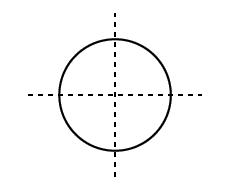QuestionAnswers

# Identify how many lines of symmetry drawn are there in the given 2D figure?Verified
Hint: In a Circle, A line (drawn at any angle) that goes through its center is a Line of Symmetry. So a Circle has infinite Lines of Symmetry. It is not necessary that all the figures possess a line or lines of symmetry in different figures. Figures may have no line of symmetry sometimes or they may have $1,{\text{ }}2,{\text{ }}3,{\text{ }}4{\text{ }} \ldots \infty$ number of lines of symmetry as a circle is having Infinite lines of symmetry.